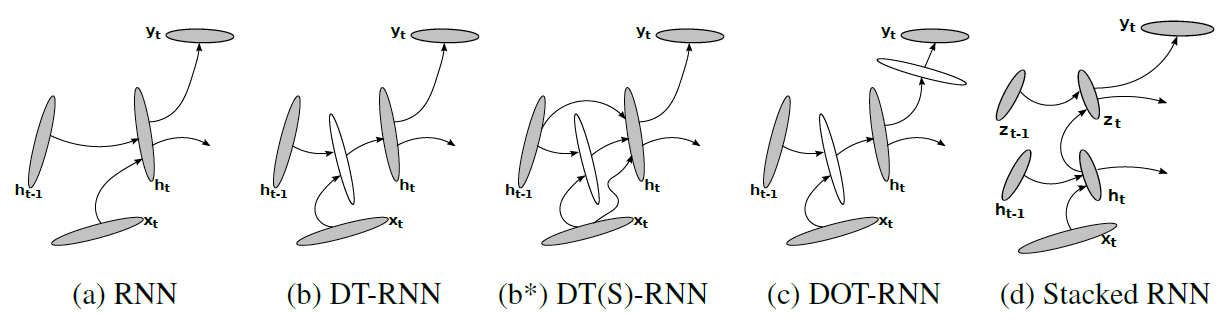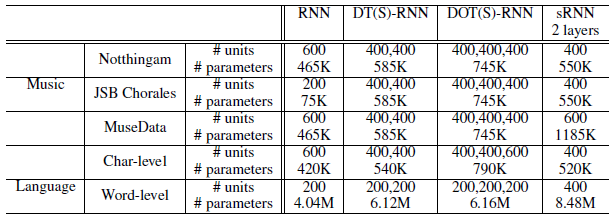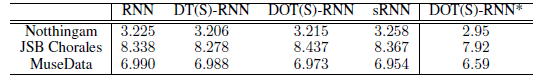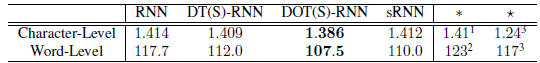# Brief Review — How to Construct Deep Recurrent Neural Networks

## Design Deep RNN Without Using Memory-Based LSTM/GRU

How to Construct Deep Recurrent Neural Networks
DT-RNN, DOT-RNN, sRNN, by Université de Montréal
2014 ICLR, Over 1000 Citations (Sik-Ho Tsang @ Medium)
Recurrent Neural Network, RNN, Sequence Model, Language Model

• At that moment, there was a bottleneck of how to construct a efficient deep RNN when LSTM and GRU those gated networks are not used.
• Non-linear layers are added to make RNN deeper.
• This is a paper from Bengio’s research group.

# Outline

1. Proposed DT-RNN, DOT-RNN, sRNN
2. Results

# 1. Proposed DT-RNN, DOT-RNN, sRNN

## 1.1. Proposed Deep RNN VariantsFour different recurrent neural networks (RNN). (a) A conventional RNN. (b) Deep Transition (DT) RNN. (b*) DT-RNN with shortcut connections © Deep Transition, Deep Output (DOT) RNN. (d) Stacked RNN (The figure is quite free style, lol.)
• (a) Conventional RNN: State ht and output yt are generated as follows:
• where fh and fo are a state transition function and an output function.
• Nonlinear function, such as a logistic sigmoid function or a hyperbolic tangent function, is used.
• (b) Deep Transition RNN (DT-RNN): A highly nonlinear transition is modeled by an MLP (white color):
• (b*) DT(S)-RNN: DT-RNN with skip connection.
• (c) DOT-RNN: One more MLP layer is added at the output:
• (d) Stacked RNN: One more RNN is added on top of the RNN:

## 1.2. Model VariantsThe sizes of the trained models
• The above table shows the RNN models dedicated to music prediction and language model.
• Gradient clipping, in SGD+CR, is used.

# 2. Results

## 2.1. Polyphonic Music PredictionThe log-probabilities on the test set
• The best results obtained by the DT(S)-RNNs on Notthingam and JSB Chorales are close to, but worse than the result obtained by RNNs trained with the technique of fast dropout (FD) which are 3.09 and 8.01, respectively.

## 2.2. Language Model / Word PredictionThe perplexities on the test set
• *: The previous/current state-of-the-art results obtained with shallow RNNs. : The previous/current state-of-the-art results obtained with RNNs having LSTM units.
• Deep RNNs (DT(S)-RNN, DOT(S)-RNN and sRNN) outperform the conventional, shallow RNN significantly.
• The results by both the DOT(S)-RNN and the sRNN for word-level modeling surpassed the previous best performance achieved by an RNN with 1000 long short-term memory (LSTM) units.

(This paper has been in my hard drive for many years, but haven’t read yet, until now…)

## Reference

[2014 ICLR] [DT-RNN, DOT-RNN, sRNN]
How to Construct Deep Recurrent Neural Networks

## Language Model / Sequence Model

20072014 … [DT-RNN, DOT-RNN, sRNN] … 2020 [ALBERT] [GPT-3] [T5] [Pre-LN Transformer] [MobileBERT] [TinyBERT]

--

--